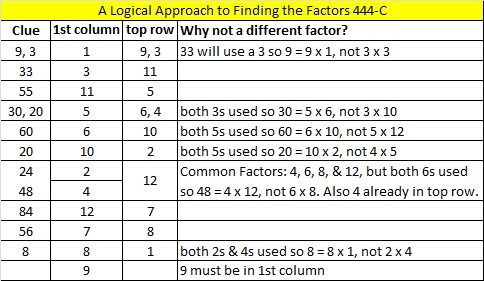# 299 and Level 4

• 299 is a composite number.
• Prime factorization: 299 = 13 x 23
• The exponents in the prime factorization are 1 and 1. Adding one to each and multiplying we get (1 + 1)(1 + 1) = 2 x 2 = 4. Therefore 299 has exactly 4 factors.
• Factors of 299: 1, 13, 23, 299
• Factor pairs: 299 = 1 x 299 or 13 x 23
• 299 has no square factors that allow its square root to be simplified. √299 ≈ 17.292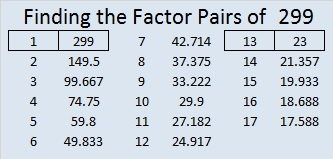Warning: The logic needed to solve this Level 4 puzzle is complicated.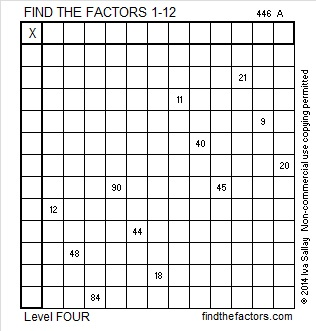Print the puzzles or type the factors on this excel file: 12 Factors 2014-11-17# 298 and Level 3

• 298 is a composite number.
• Prime factorization: 298 = 2 x 149
• The exponents in the prime factorization are 1 and 1. Adding one to each and multiplying we get (1 + 1)(1 + 1) = 2 x 2 = 4. Therefore 298 has exactly 4 factors.
• Factors of 298: 1, 2, 149, 298
• Factor pairs: 298 = 1 x 298 or 2 x 149
• 298 has no square factors that allow its square root to be simplified. √298 ≈ 17.263Can you fill out this 1 – 12 multiplication table when you’re only given twelve clues?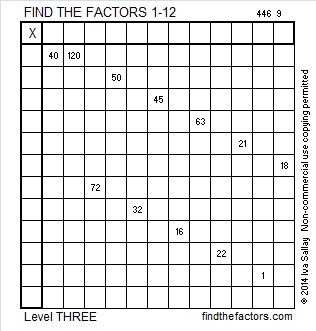Print the puzzles or type the factors on this excel file: 12 Factors 2014-11-17

A Logical Approach to FIND THE FACTORS: Find the column or row with two clues and find their common factor. Write the corresponding factors in the factor column (1st column) and factor row (top row).  Because this is a level three puzzle, you have now written a factor at the top of the factor column. Continue to work from the top of the factor column to the bottom, finding factors and filling in the factor column and the factor row one cell at a time as you go.# 297 and Level 2

• 297 is a composite number.
• Prime factorization: 297 = 3 x 3 x 3 x 11 which can be written 297 = 3³ x 11
• The exponents in the prime factorization are 3 and 1. Adding one to each and multiplying we get (3 + 1)(1 + 1) = 4 x 2 = 8. Therefore 297 has exactly 8 factors.
• Factors of 297: 1, 3, 9, 11, 27, 33, 99, 297
• Factor pairs: 297 = 1 x 297, 3 x 99, 9 x 33, or 11 x 27
• Taking the factor pair with the largest square number factor, we get √297 = (√9)(√33) = 3√33 ≈ 17.234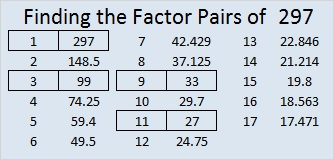This multiplication table has only 14 clues. Is that enough to complete it?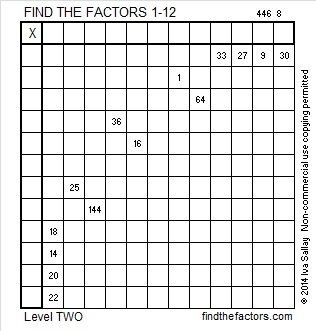Print the puzzles or type the factors on this excel file: 12 Factors 2014-11-17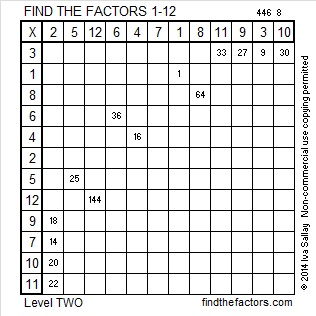# 296 and Level 1

• 296 is a composite number.
• Prime factorization: 296 = 2 x 2 x 2 x 37 which can be written 296 = 2³ x 37
• The exponents in the prime factorization are 3 and 1. Adding one to each and multiplying we get (3 + 1)(1 + 1) = 4 x 2 = 8. Therefore 296 has exactly 8 factors.
• Factors of 296: 1, 2, 4, 8, 37, 74, 148, 296
• Factor pairs: 296 = 1 x 296, 2 x 148, 4 x 74, or 8 x 37
• Taking the factor pair with the largest square number factor, we get √296 = (√4)(√74) = 2√74 ≈ 17.2046505Although I came home from the hospital on Sunday, I haven’t felt like spending much time on the computer reading or writing anything. Full recovery will be another five weeks, but hopefully I’ll start feeling better in less time.  I do feel better today than I did on Sunday.

Print the puzzles or type the factors on this excel file: 12 Factors 2014-11-17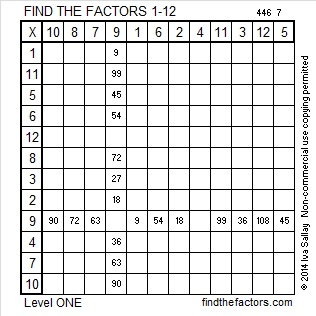# 295 and Level 6

• 295 is a composite number.
• Prime factorization: 295 = 5 x 59
• The exponents in the prime factorization are 1 and 1. Adding one to each and multiplying we get (1 + 1)(1 + 1) = 2 x 2 = 4. Therefore 295 has exactly 4 factors.
• Factors of 295: 1, 5, 59, 295
• Factor pairs: 295 = 1 x 295 or 5 x 59
• 295 has no square factors that allow its square root to be simplified. √295 ≈ 17.176These eleven clues could be pretty tricky!Print the puzzles or type the factors on this excel file: 10 Factors 2014-11-10# 294 and Level 5

• 294 is a composite number.
• Prime factorization: 294 = 2 x 3 x 7 x 7, which can be written 294 = 2 x 3 x (7^2)
• The exponents in the prime factorization are 1, 1 and 2. Adding one to each and multiplying we get (1 + 1)(1 + 1)(2 + 1) = 2 x 2 x 3 = 12. Therefore 294 has exactly 12 factors.
• Factors of 294: 1, 2, 3, 6, 7, 14, 21, 42, 49, 98, 147, 294
• Factor pairs: 294 = 1 x 294, 2 x 147, 3 x 98, 6 x 49, 7 x 42, or 14 x 21
• Taking the factor pair with the largest square number factor, we get √294 = (√6)(√49) = 7√6 ≈ 17.146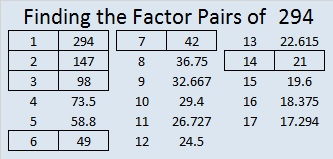Can you fill out this multiplication table that has only eleven clues?Print the puzzles or type the factors on this excel file: 10 Factors 2014-11-10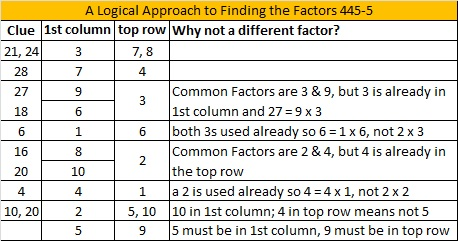# 292 and Level 3

• 292 is a composite number.
• Prime factorization: 292 = 2 x 2 x 73, which can be written 292 = 2² x 73
• The exponents in the prime factorization are 2 and 1. Adding one to each and multiplying we get (2 + 1)(1 + 1) = 3 x 2  = 6. Therefore 292 has exactly 6 factors.
• Factors of 292: 1, 2, 4, 73, 146, 292
• Factor pairs: 292 = 1 x 292, 2 x 146, or 4 x 73
• Taking the factor pair with the largest square number factor, we get √292 = (√4)(√73) = 2√73 ≈ 17.088.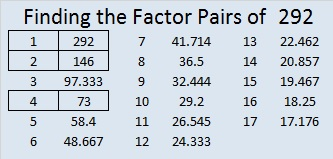Can you fill out this multiplication table that has only ten clues?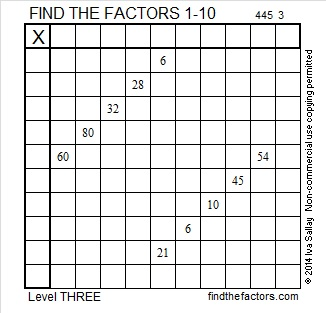Print the puzzles or type the factors on this excel file: 10 Factors 2014-11-10

A Logical Approach to FIND THE FACTORS: Find the column or row with two clues and find their common factor. Write the corresponding factors in the factor column (1st column) and factor row (top row).  Because this is a level three puzzle, you have now written a factor at the top of the factor column. Continue to work from the top of the factor column to the bottom, finding factors and filling in the factor column and the factor row one cell at a time as you go.# 291 and Level 2

• 291 is a composite number.
• Prime factorization: 291 = 3 x 97
• The exponents in the prime factorization are 1 and 1. Adding one to each and multiplying we get (1 + 1)(1 + 1) = 2 x 2 = 4. Therefore 291 has exactly 4 factors.
• Factors of 291: 1, 3, 97, 291
• Factor pairs: 291 = 1 x 291 or 3 x 97
• 291 has no square factors that allow its square root to be simplified. √291 ≈ 17.059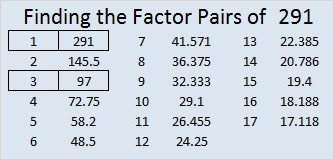Can you complete this multiplication table if you’re given just twelve clues?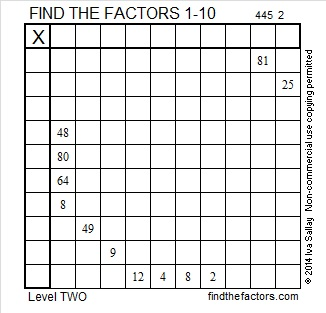Print the puzzles or type the factors on this excel file: 10 Factors 2014-11-10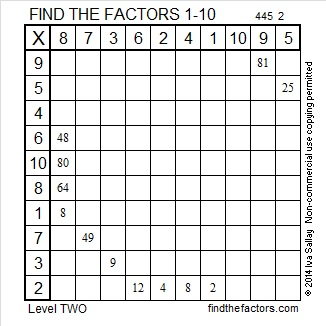# 290 and Level 1

• 290 is a composite number.
• Prime factorization: 290 = 2 x 5 x 29
• The exponents in the prime factorization are 1, 1, and 1. Adding one to each and multiplying we get (1 + 1)(1 + 1)(1 + 1) = 2 x 2 x 2 = 8. Therefore 290 has 8 factors.
• Factors of 290: 1, 2, 5, 10, 29, 58, 145, 290
• Factor pairs: 1 x 290, 2 x 145, 5 x 58, or 10 x 29
• 290 has no square factors that allow its square root to be simplified. √290 ≈ 17.029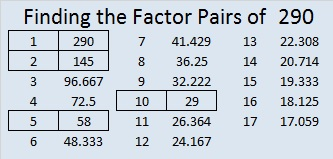Print the puzzles or type the factors on this excel file: 10 Factors 2014-11-10# 289 and Level 6

• 289 is a composite number.
• Prime factorization: 289 = 17 x 17, which can be written 289 = (17^2)
• The exponent in the prime factorization is 2. Adding one we get (2 + 1) =  3. Therefore 289 has exactly 3 factors.
• Factors of 289: 1, 17, 289
• Factor pairs: 289 = 1 x 289 or 17 x 17
• 289 is a perfect square. √289 = 17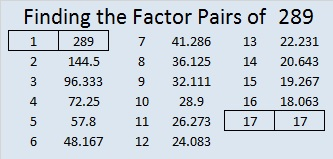1 + 3 + 5 + 7 + 9 + 11 + … + 29 + 31 + 33 = 289

Teachfurthermaths is now including Find the Factors in its list of useful links!Print the puzzles or type the factors on this excel file: 12 Factors 2014-11-03